# Is probability frequentist or Bayesian?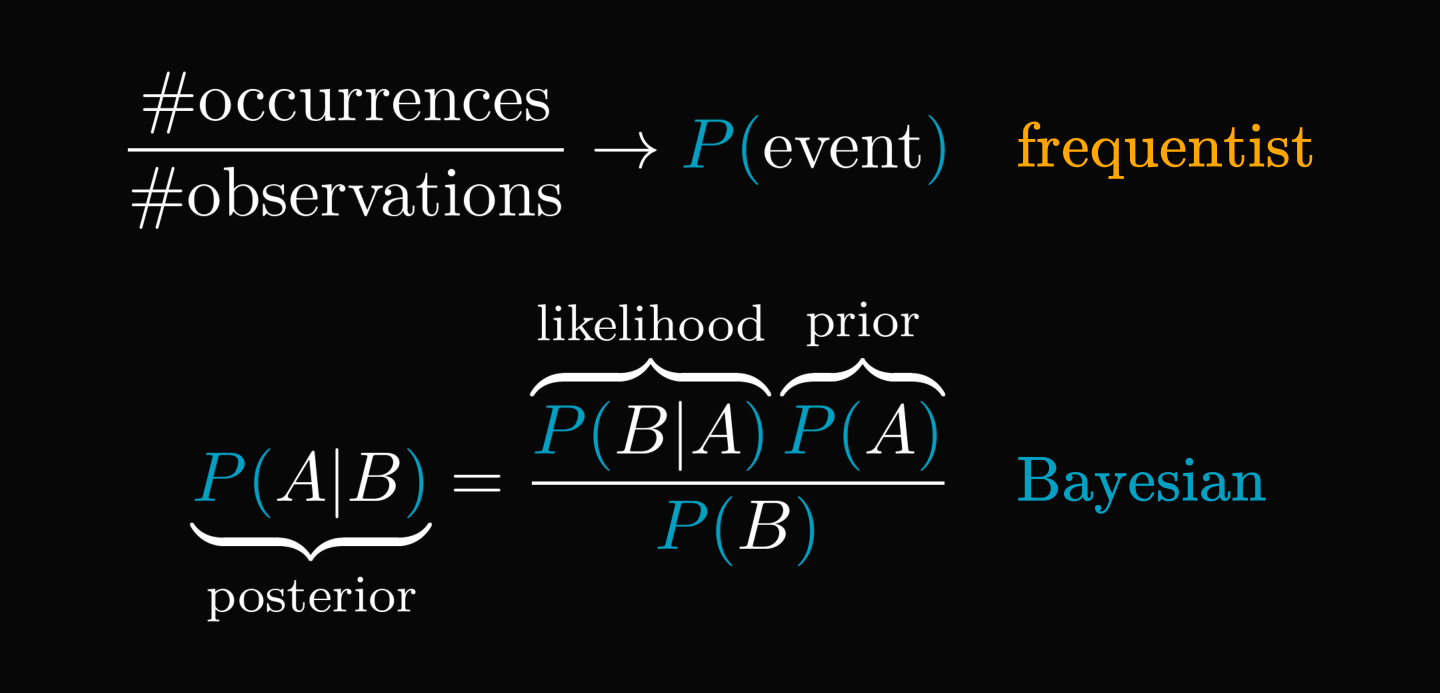The single biggest argument about statistics: is probability frequentist or Bayesian?

It's both, and I'll explain why. Buckle up. Deep-dive post below.

First, let's look at how probability behaves.

Probability quantitatively measures the likelihood of events, like rolling six with a dice. It's a number between zero and one. This is independent of interpretation.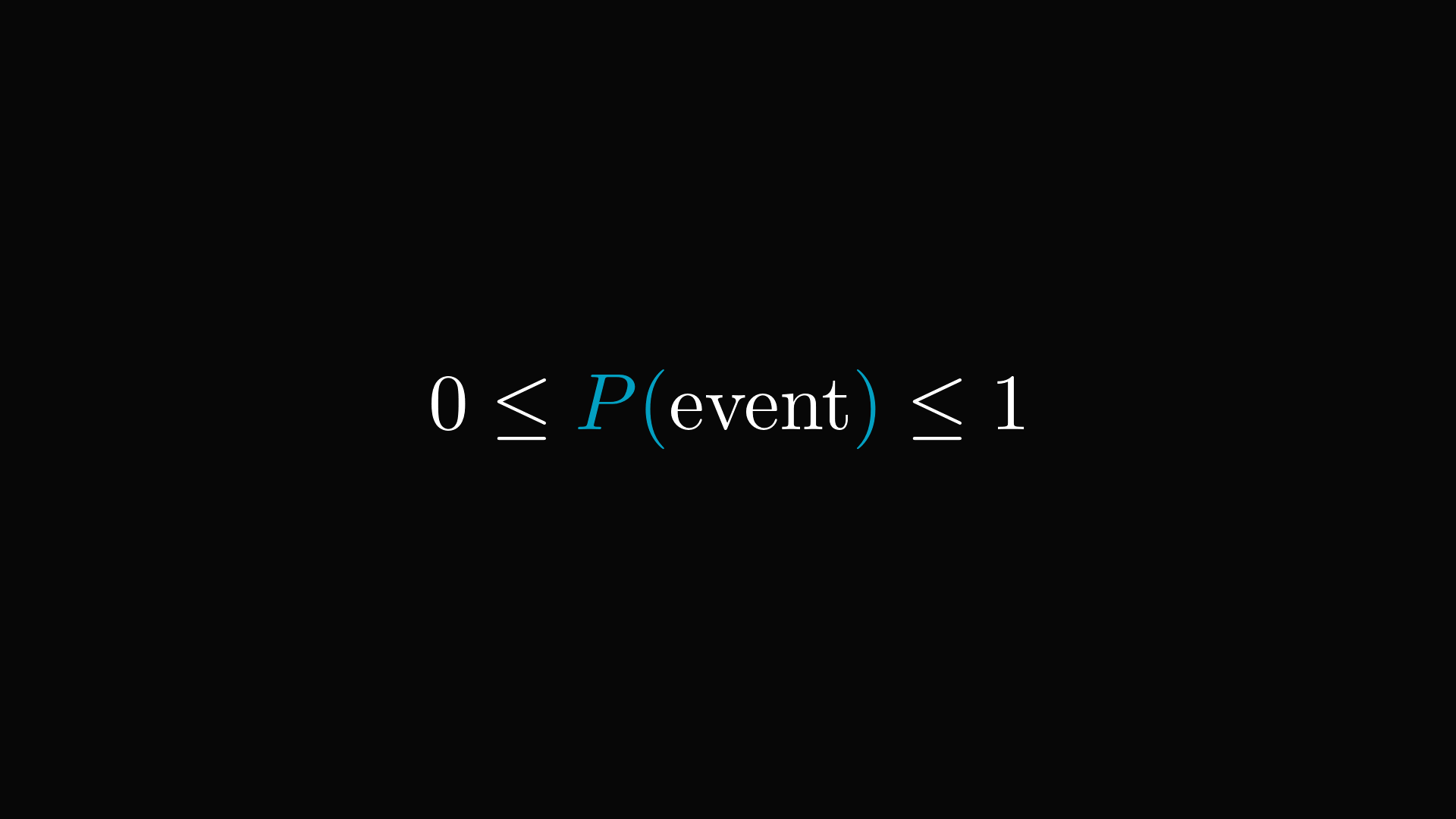In the language of mathematics, the events are formalized by sets within an event space. (The event space is also a set.)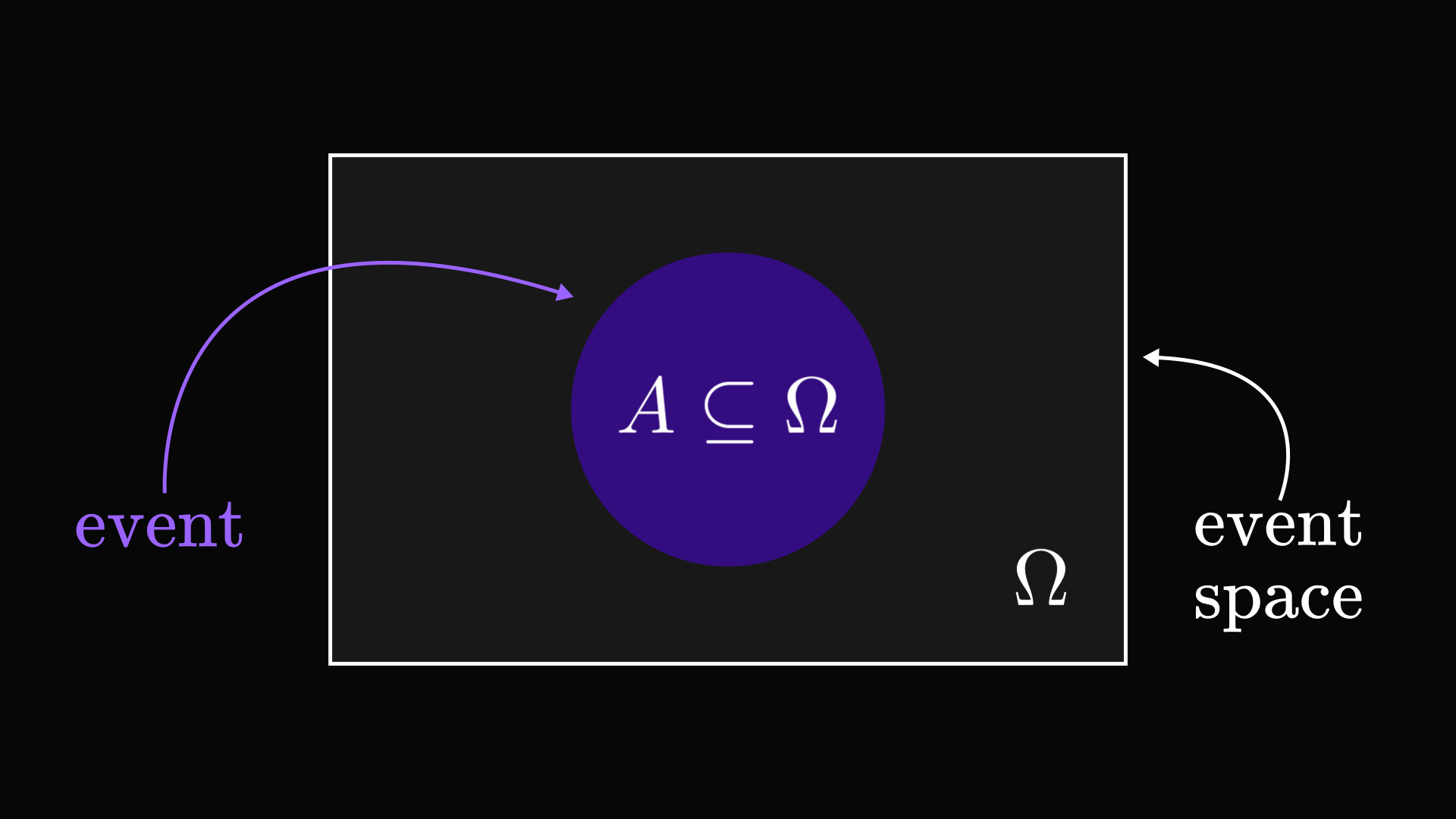The union and intersection of sets can be translated into the language of events.

The intersection of two events expresses an outcome where both events happen simultaneously. (For instance, a dice roll can be both less than 4 and an odd number. Both are proper events.)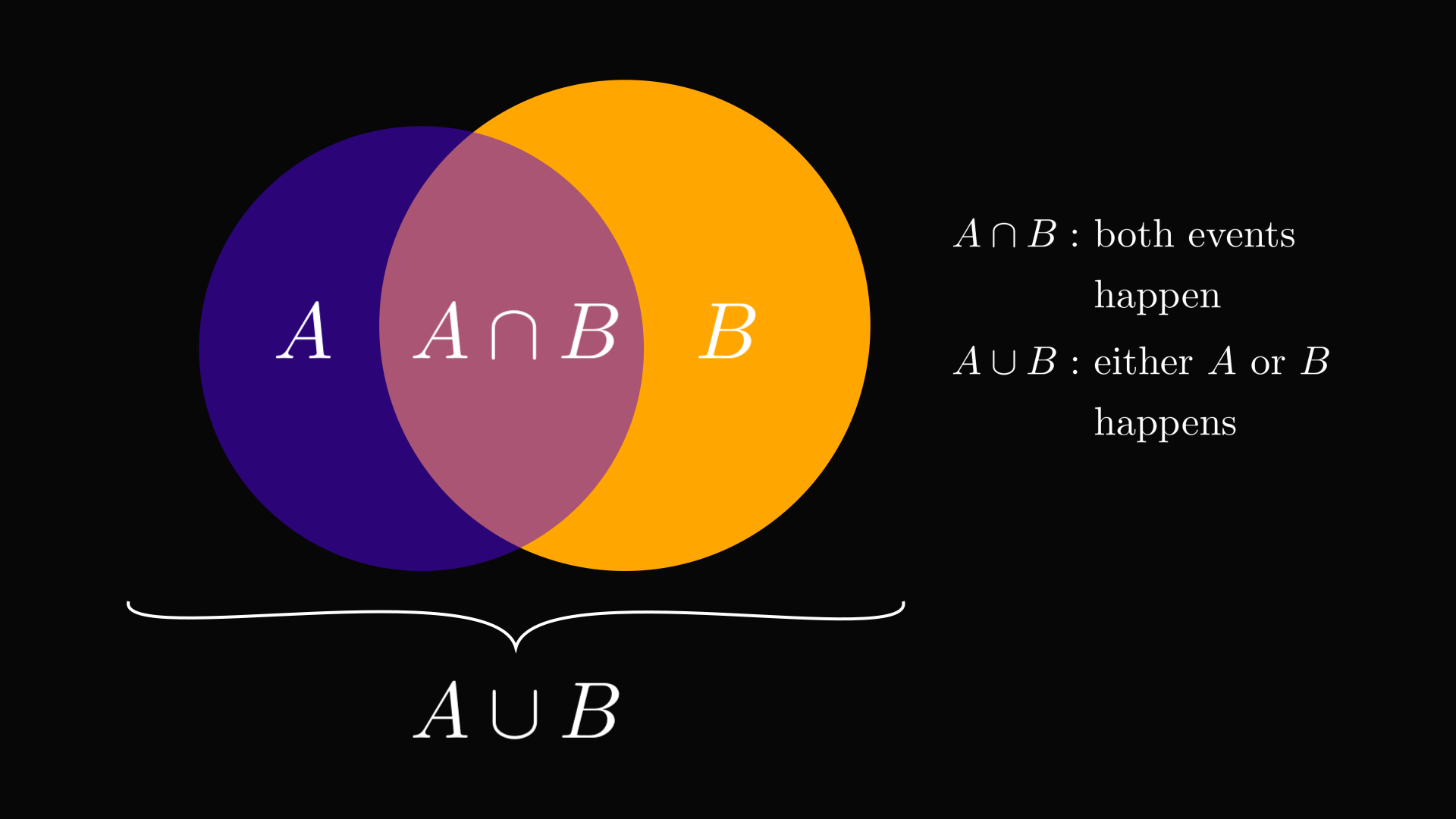We can also take the complement of an event, expressing that it does NOT happen.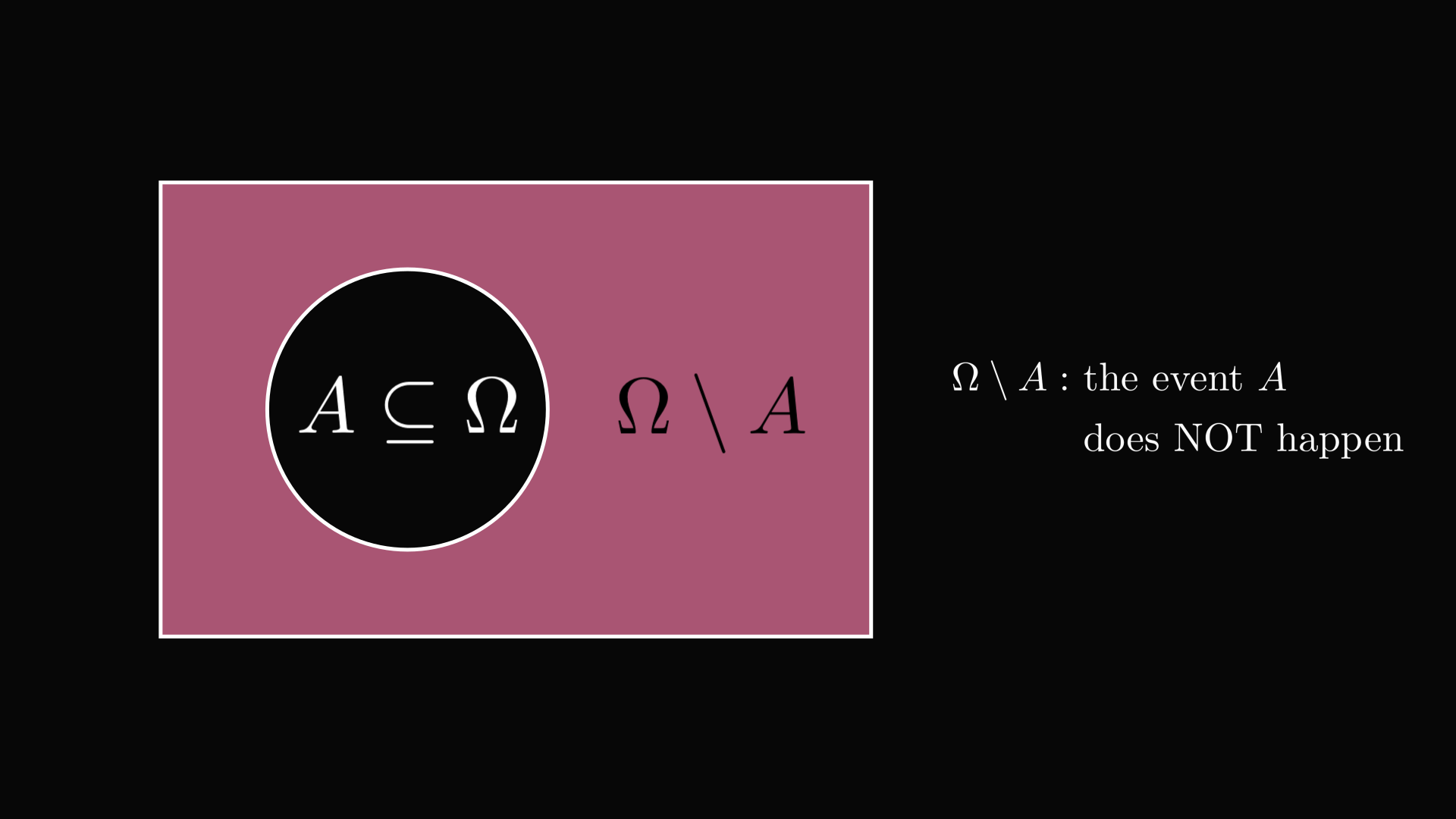So, probability is a function that takes in a set and puts out a number between 0 and 1.

There are two fundamental properties we expect from it.

First, the probability of the entire event space must be 1.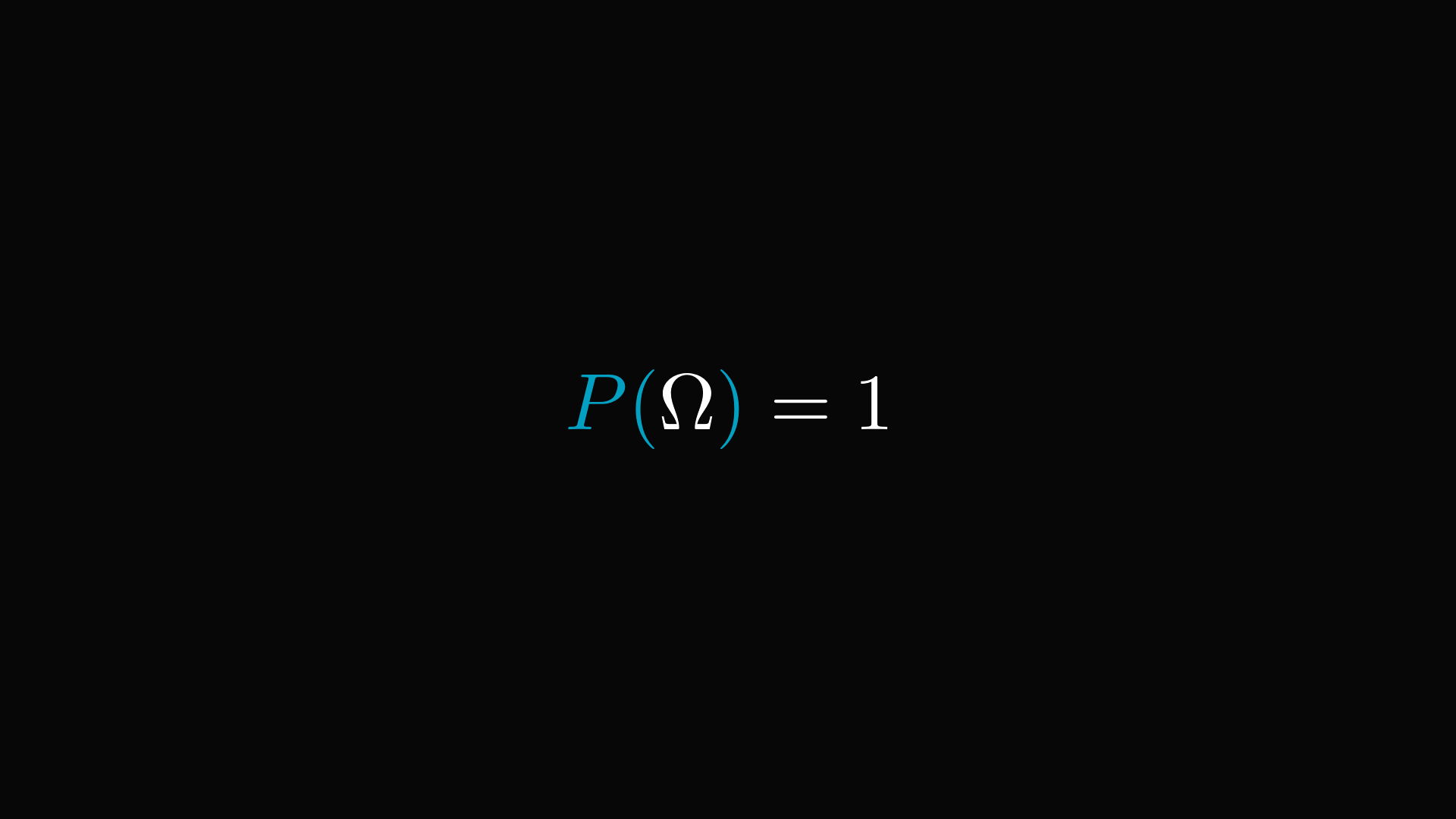Second, the probability of mutually exclusive events is the sum of their probabilities. This is intuitively clear.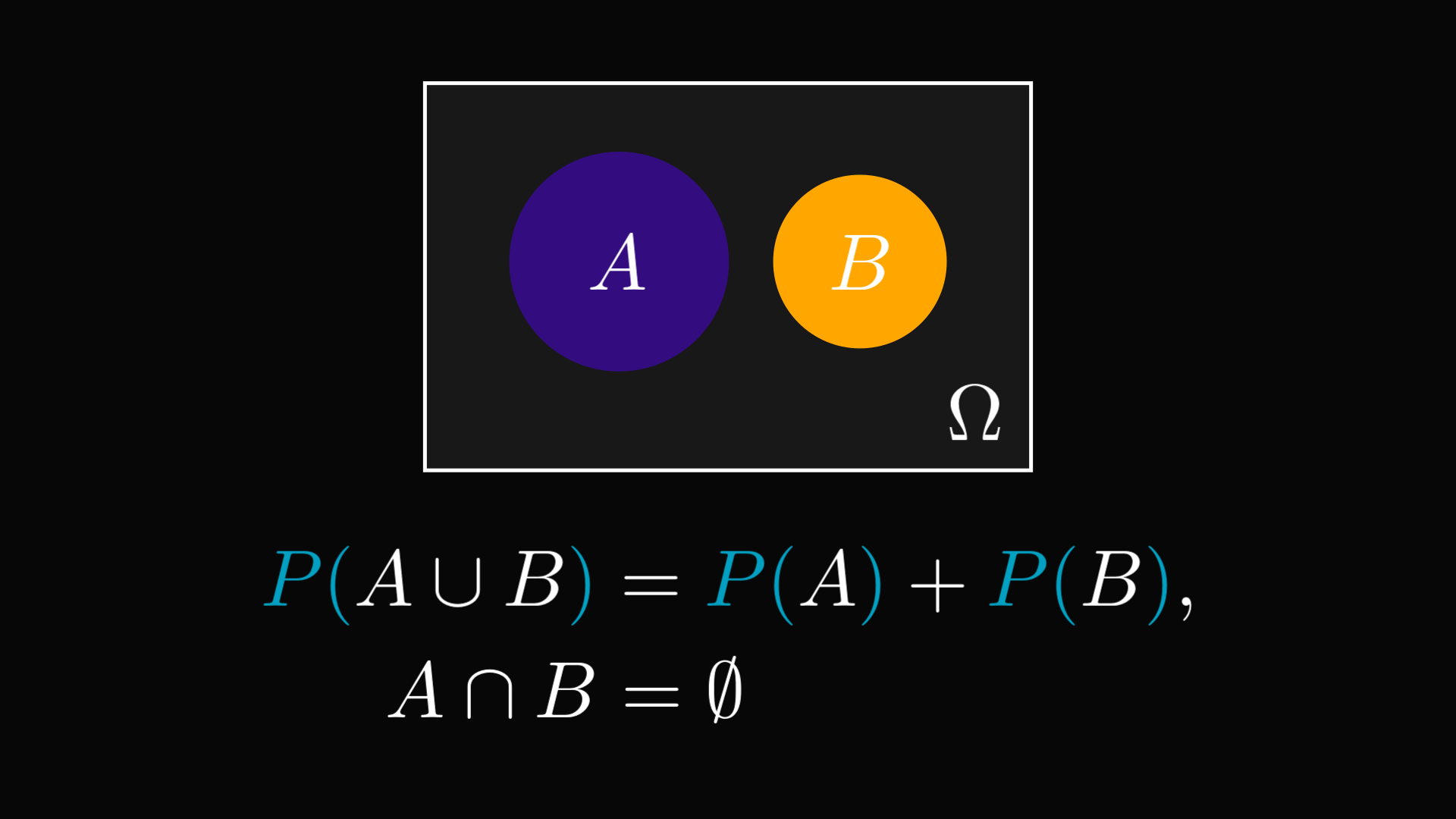In fact, this is true for any countable collection of mutually exclusive events.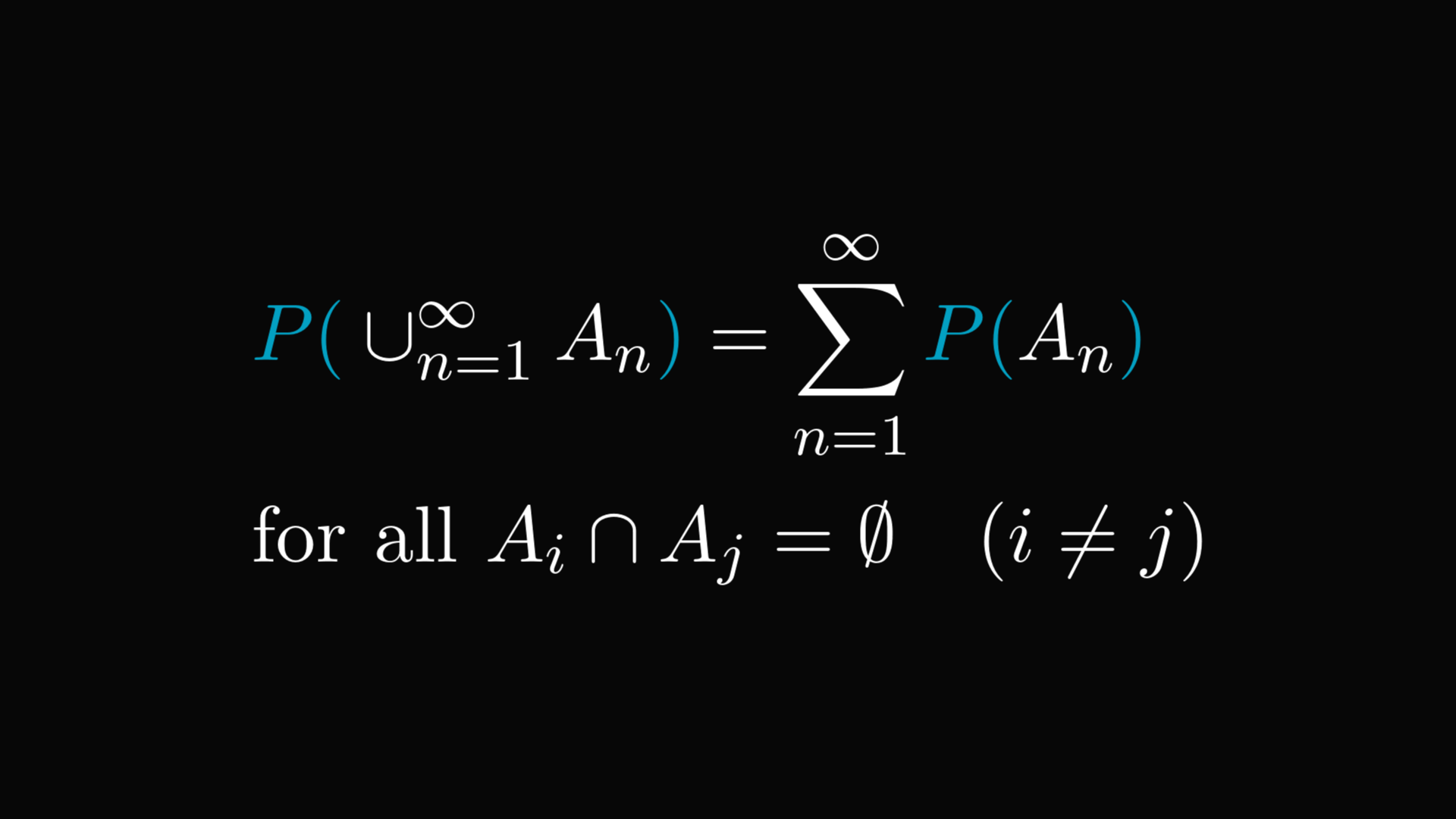These two properties can be used to define probability!

Mathematically speaking, any measure that satisfies these two axioms is a probability measure.

Let's see some examples.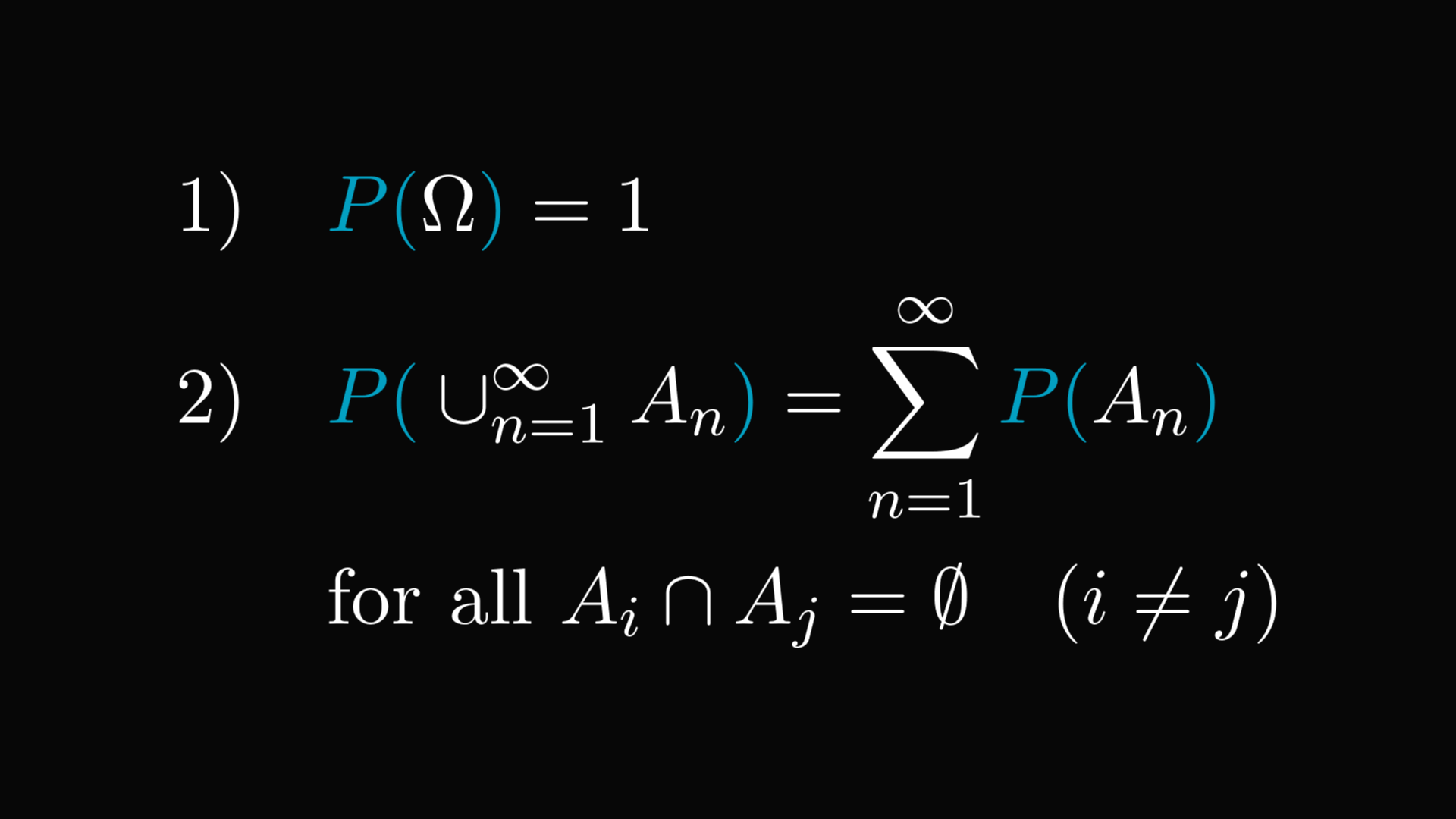1. Tossing a fair coin.

This is the simplest possible example. There are two possible outcomes, both having the same probability.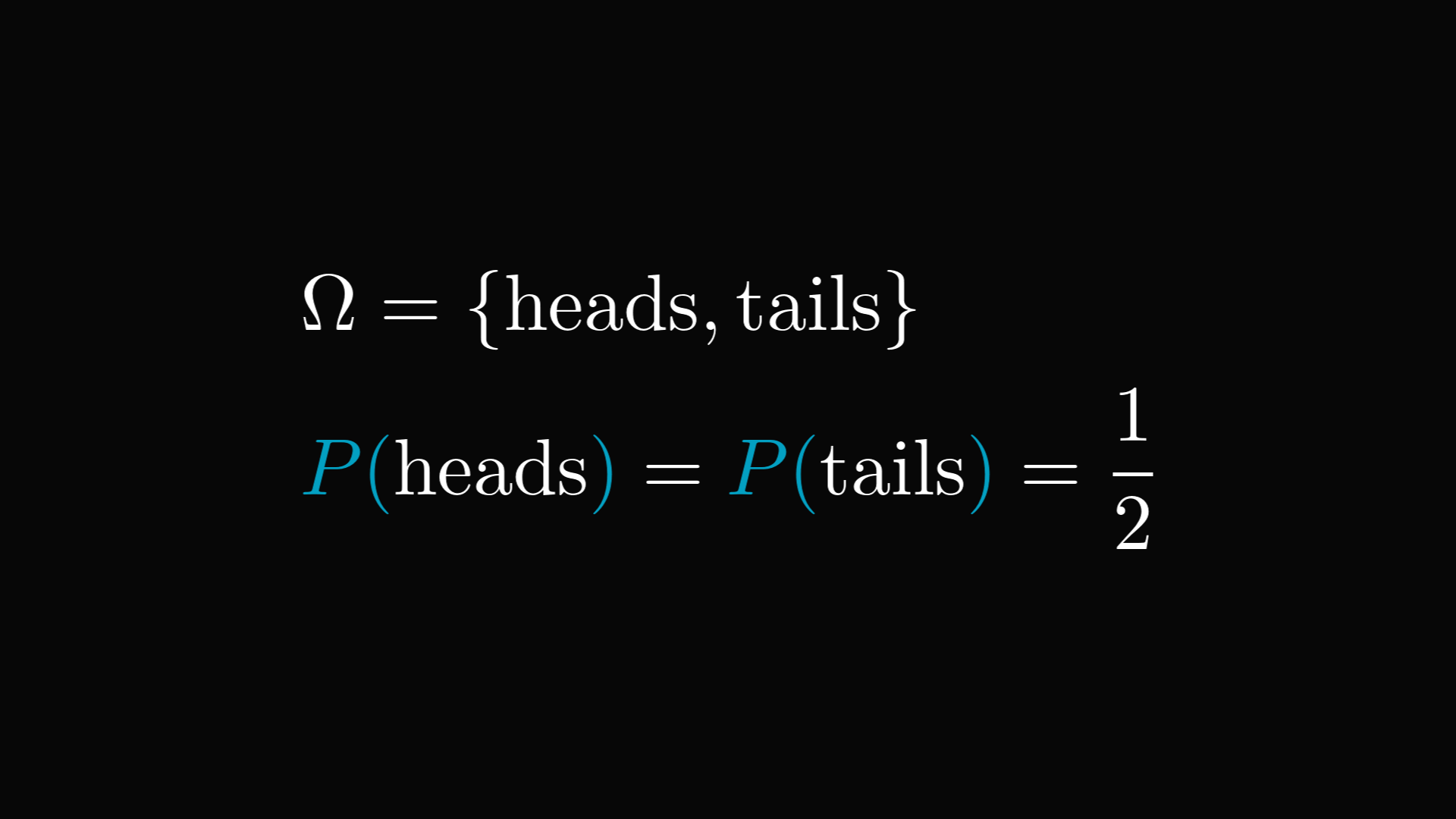1. Throwing darts.

Suppose we are throwing darts at a large wall in front of us, which is our event space. (We'll always hit the wall.)

If we throw the dart randomly, the probability of hitting a certain shape is proportional to the shape's area.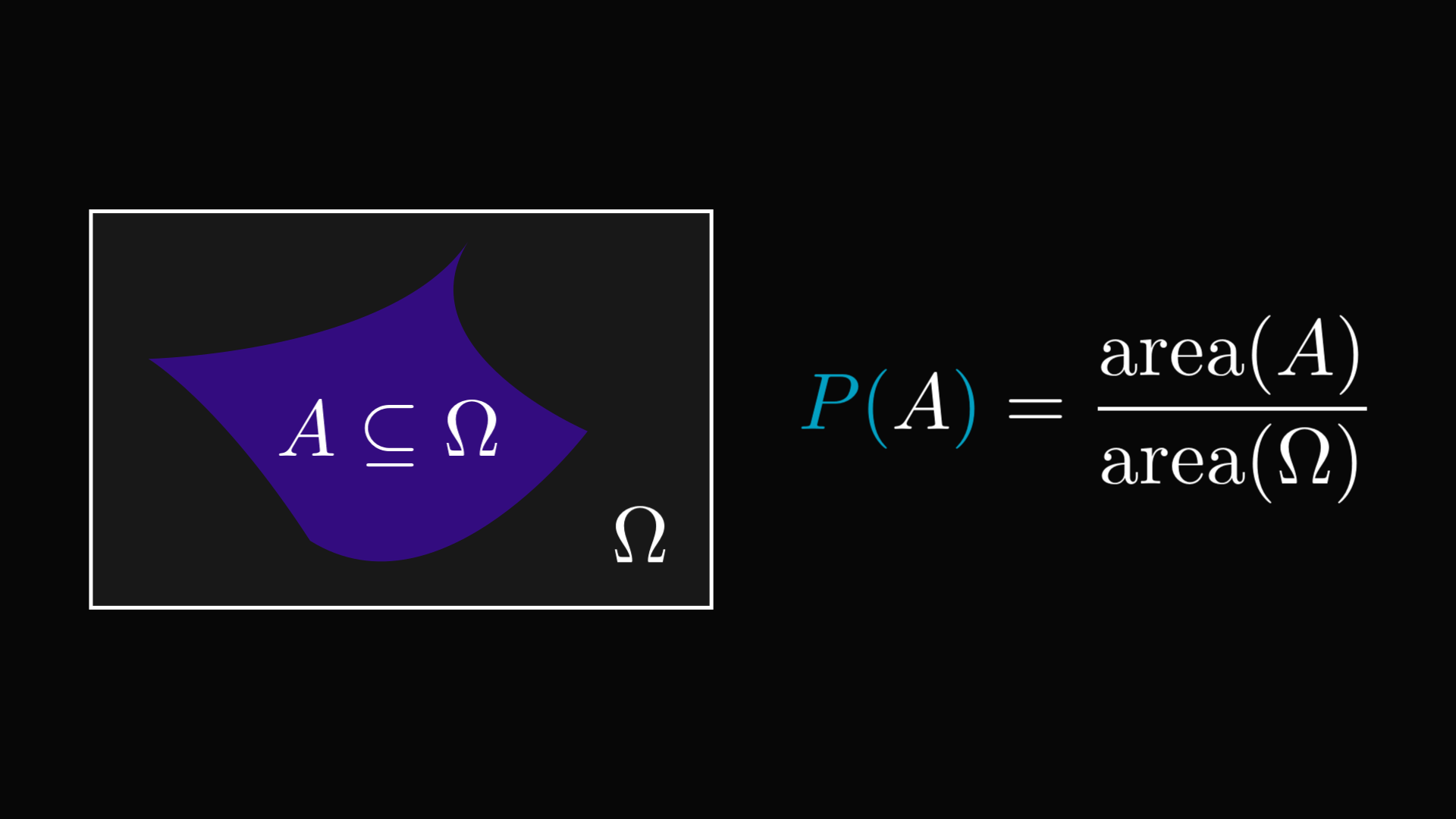Note that at this point, there is no frequentist or a Bayesian interpretation yet. Probability is a well-defined mathematical object. This concept is separated from how probabilities are assigned.

Now comes the part that has been fueling debates for decades. How can we assign probabilities? There are (at least) two schools of thought, constantly in conflict with each other.

Suppose that we repeatedly perform a single experiment, counting the number of occurrences of a given event. Say, we are tossing a coin and count the number of times it turns up heads.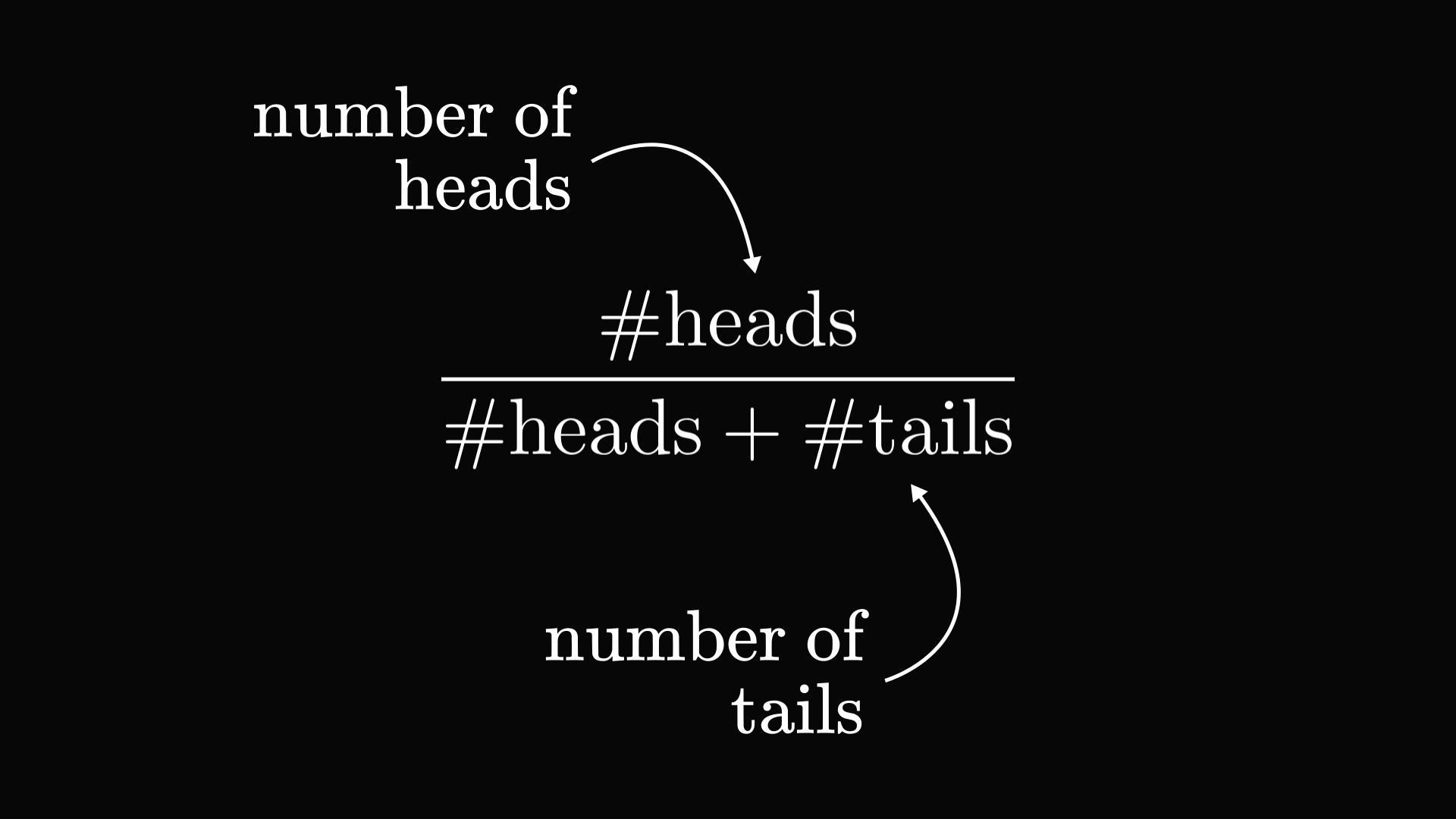The relative frequency of occurences will converge to the actual probability. (Given that the repeated experiments are independent of each other.)

This is not an interpretation of probability. This is a mathematically provable fact, independent of interpretations.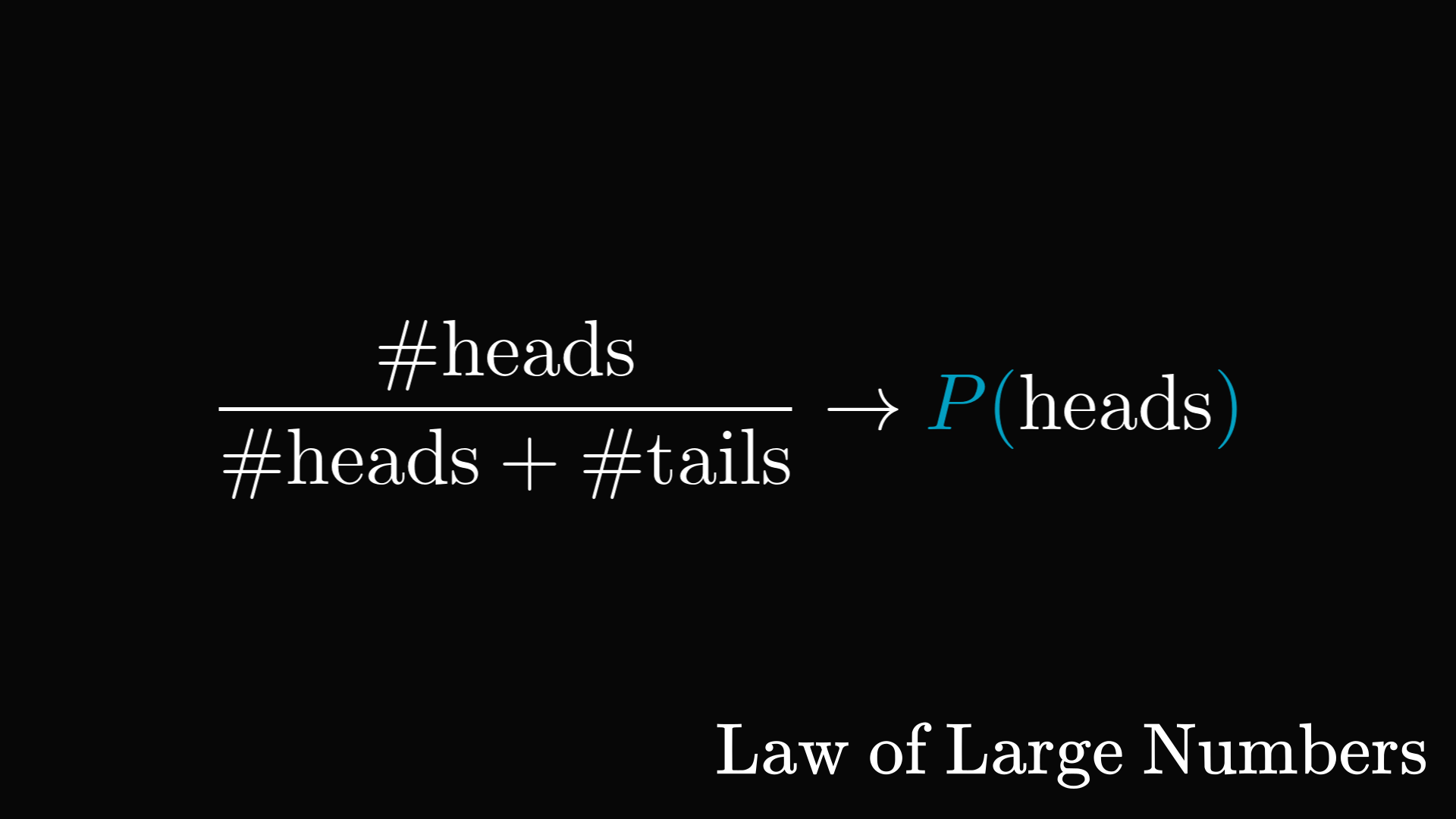Frequentists leverage this to build probabilistic models. For example, if we toss a coin $n$ times and heads come up exactly $k$ times, then the probability of heads is estimated to be $k/n$.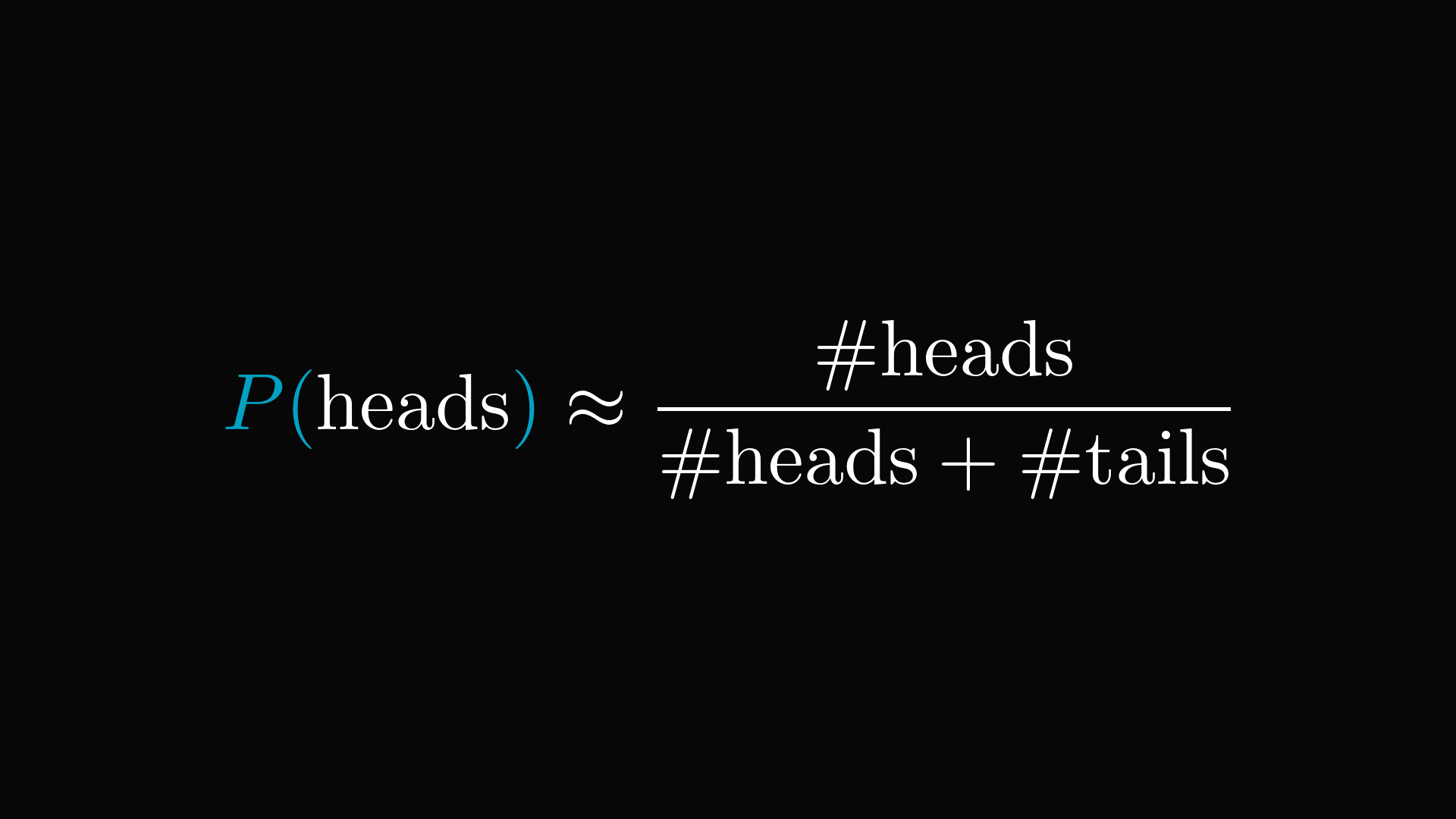On the other hand, the Bayesian school argues that such estimations are wrong, because probabilities are not absolute, but a measure of our current beliefs.

This is way too abstract, so let's elaborate.

In probabilistic models, observing certain events can influence our belief about others. For instance, if the sky is clear, the probability of rain goes down. If it is cloudy, the same probability goes up.

This is expressed in terms of conditional probabilities.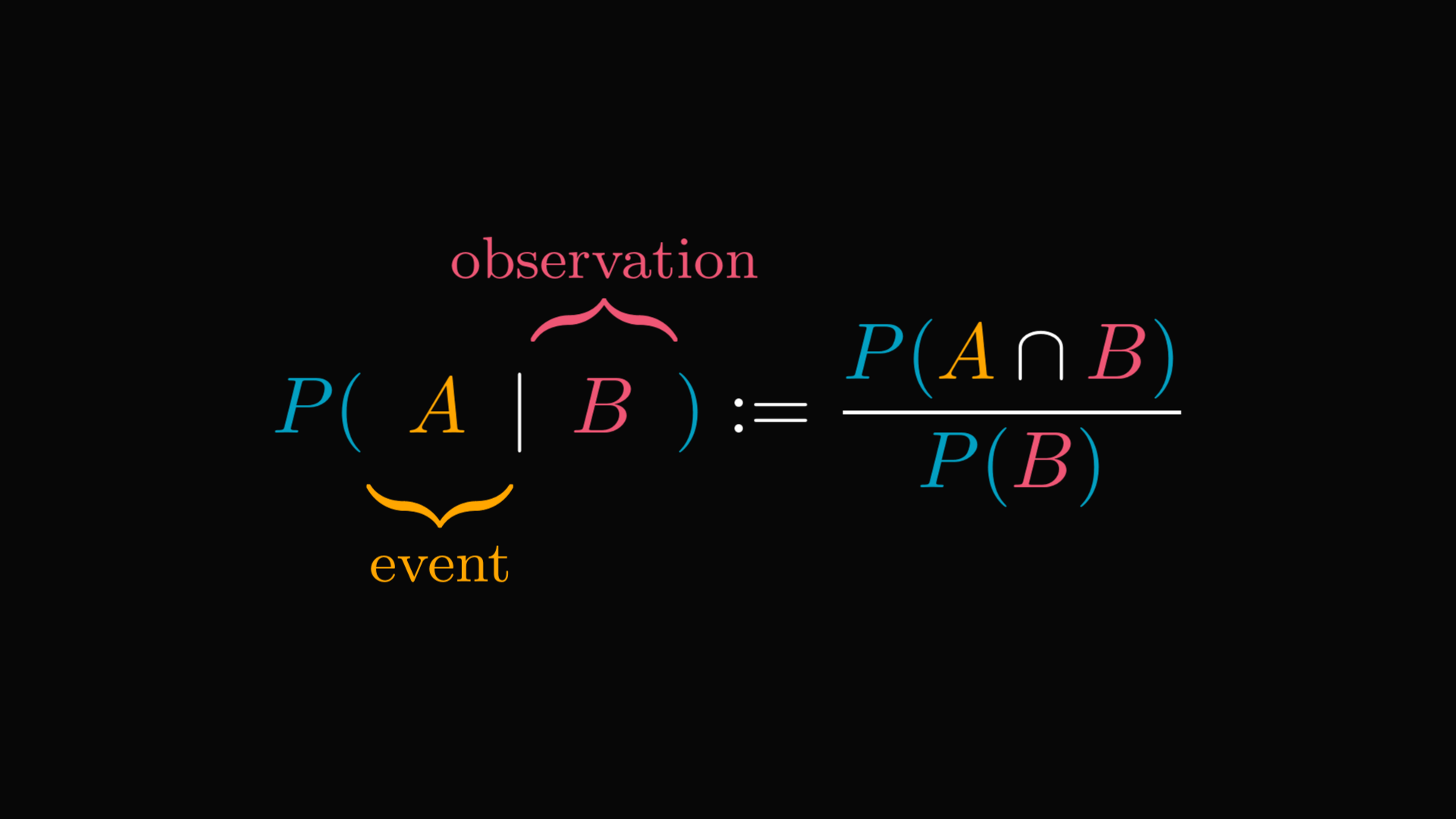In terms of conditional probabilities, this is how our intuition is expressed.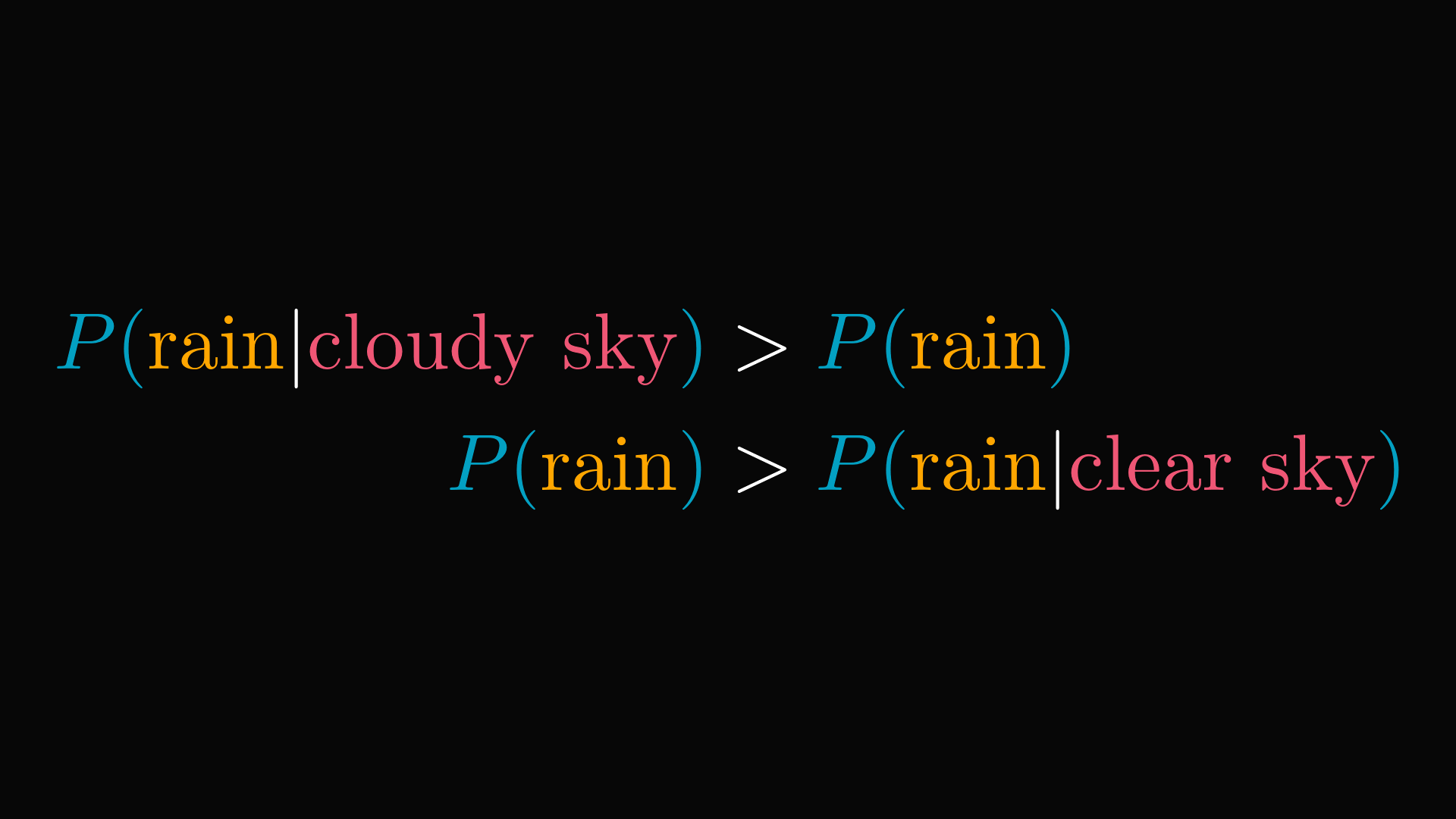Conditional probabilities allow us to update our model in light of new information.

This is called the Bayes formula, hence the terminology "Bayesian statistics". Again, this is a mathematically provable fact, not an interpretation.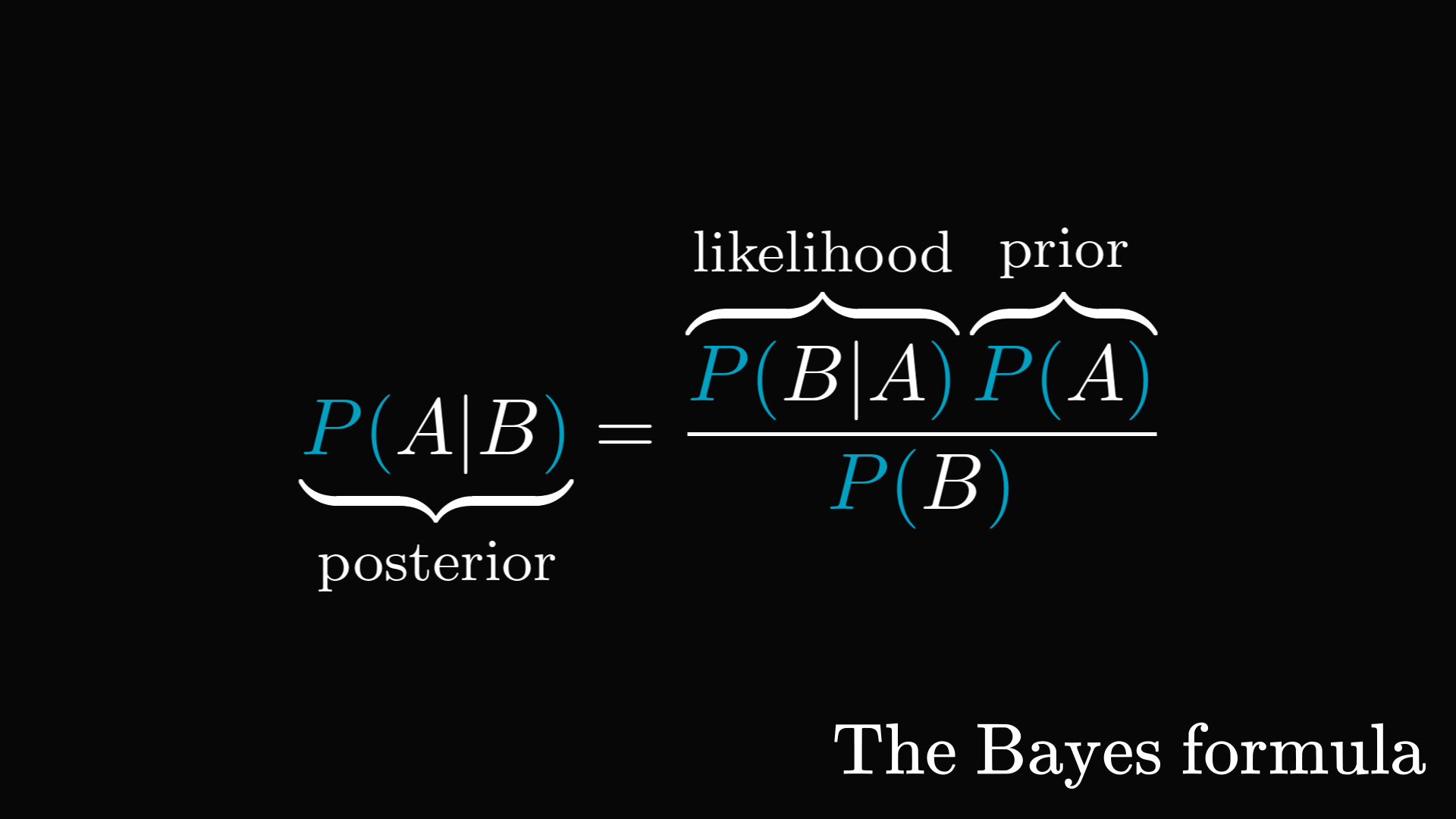Let's stick to our coin-tossing example to show how this works in practice.

Regardless of the actual probabilities, $90$ heads from $100$ tosses is a possible outcome in (almost) every case. Is the coin biased, or were we just lucky? How can we tell?

In Bayesian statistics, we treat our estimation as a random variable. This is not a simple probability, but a probability distribution or density.For instance, if we know absolutely nothing about our coin, we assume this to be uniform. This is called the prior.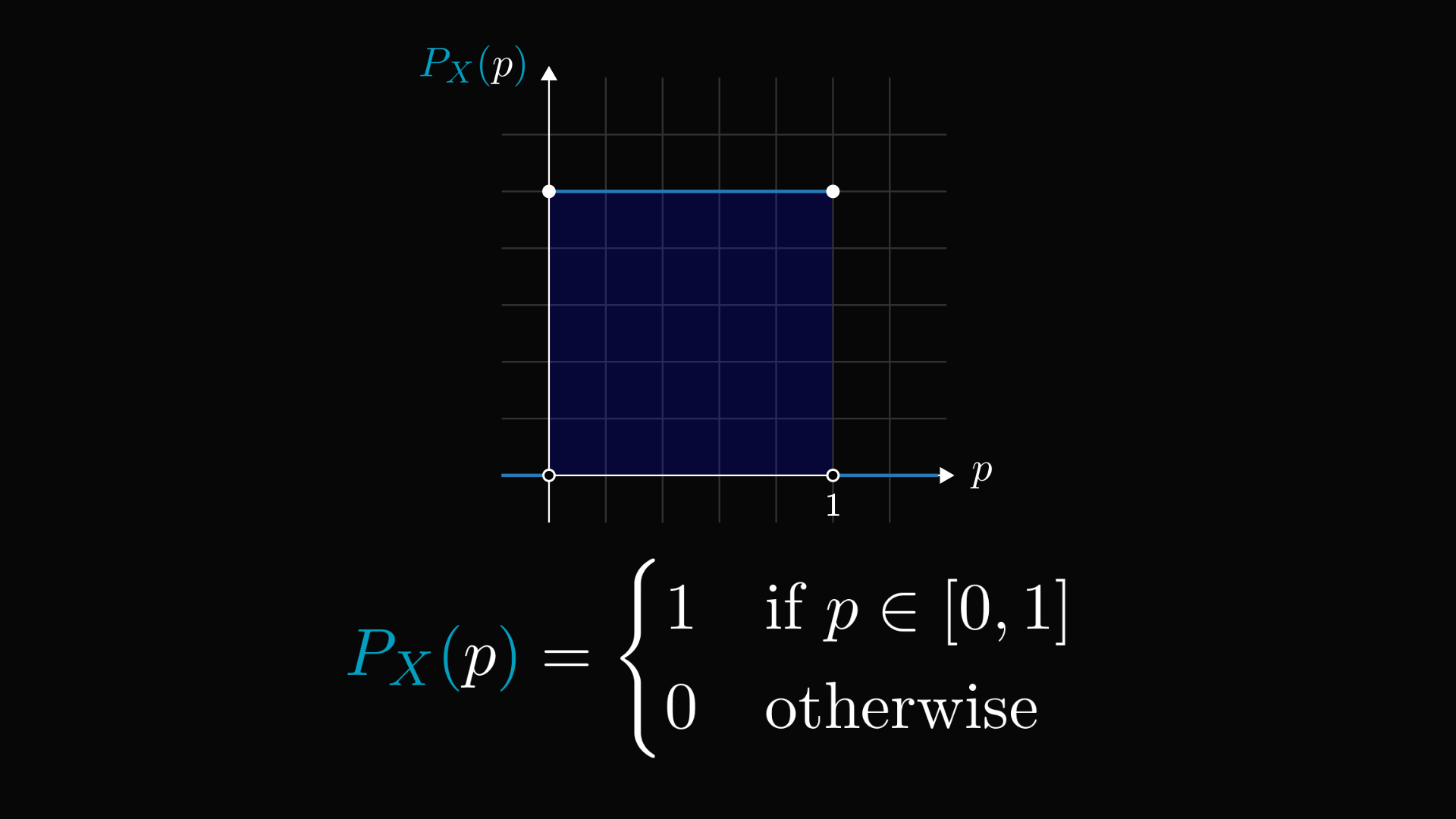What we want is to include the experimental observations in our estimation, which is expressed in terms of conditional probabilities.

This is called the posterior estimation.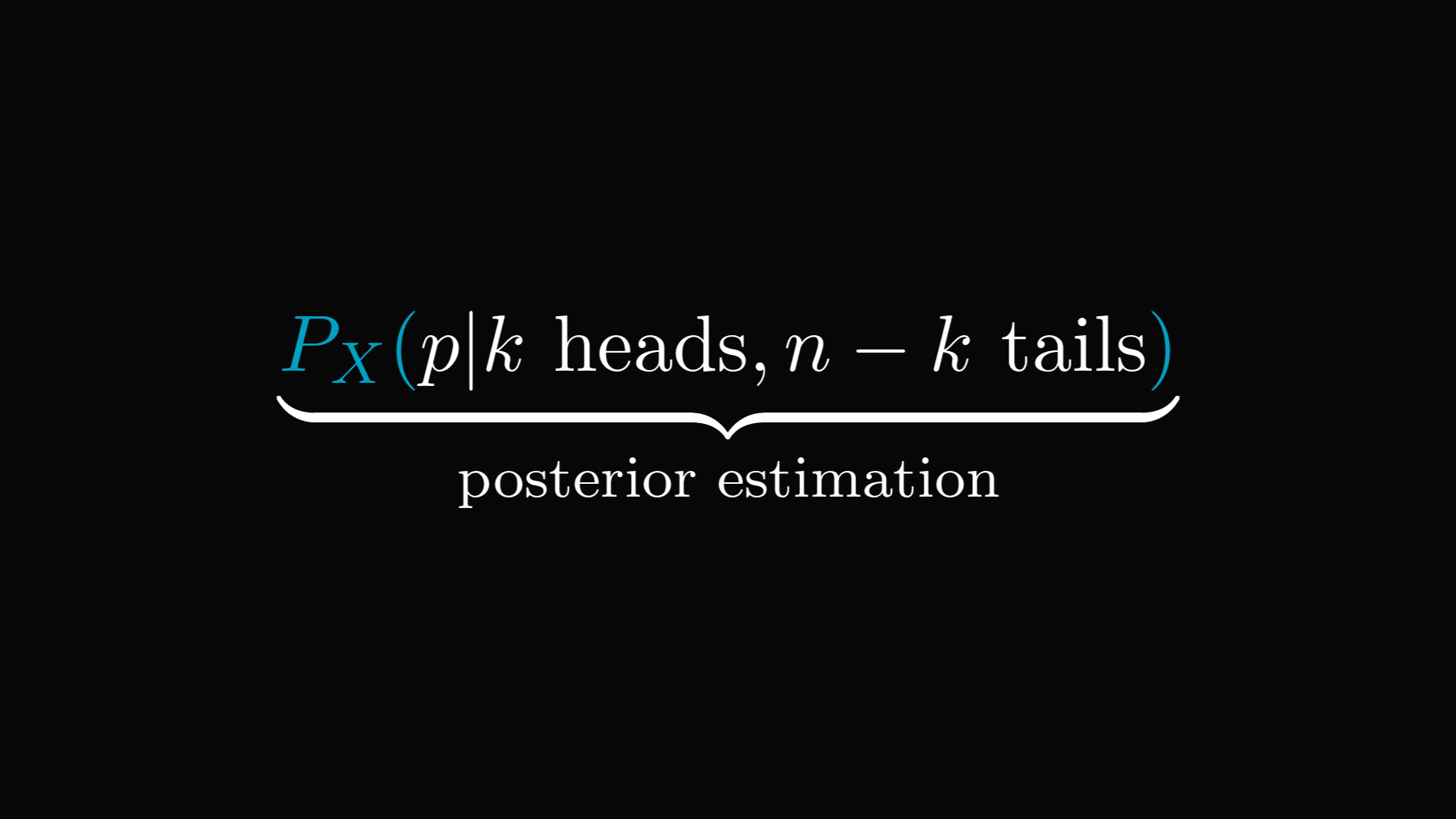The Bayes formula connects the prior and the likelihood to the posterior. Don't worry if this seems complex. We'll unravel it term by term.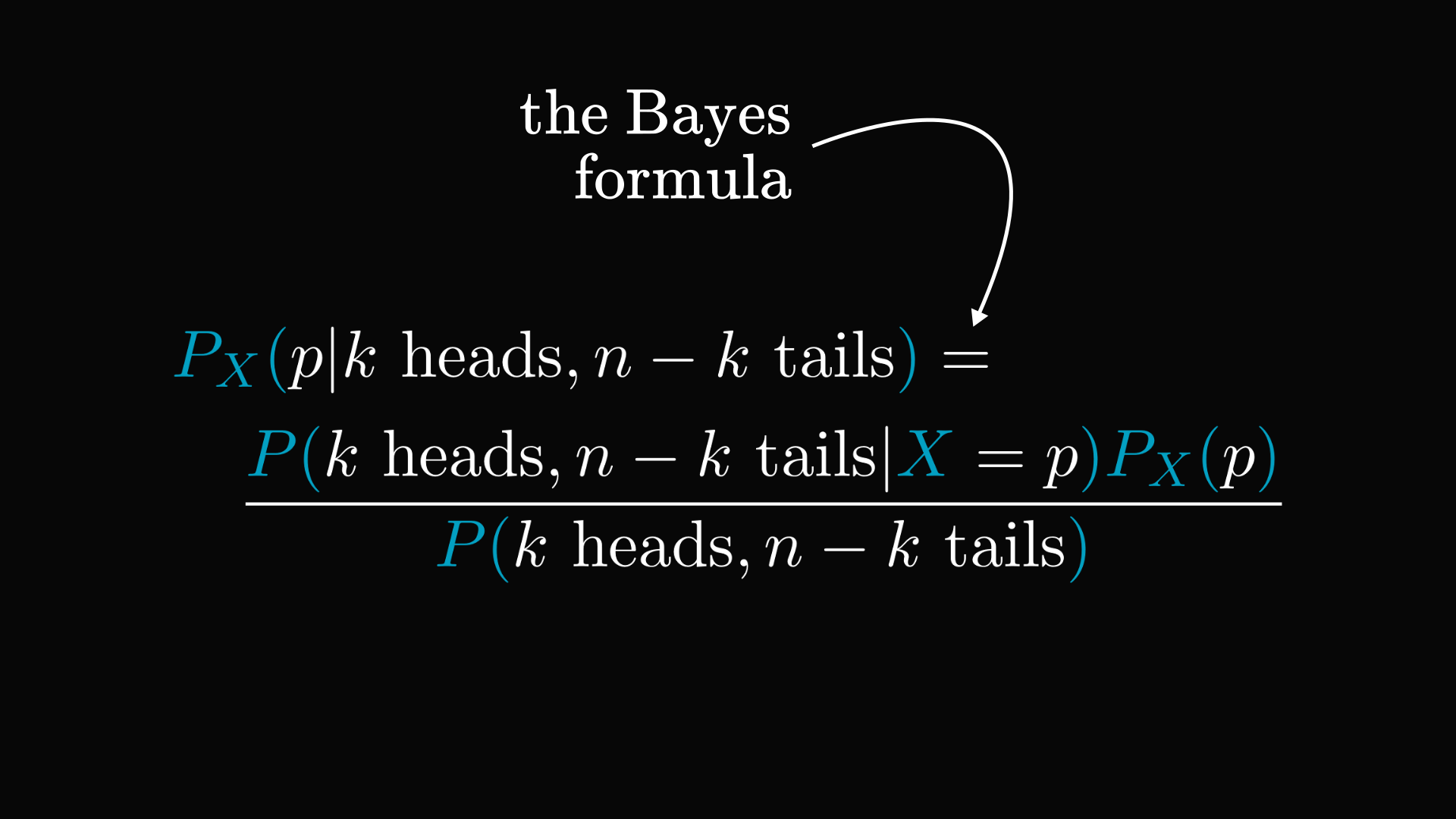There are three terms on the right side: the likelihood, the prior, and the denominator.

As the denominator is not dependent on $X$, we can omit it without any complications.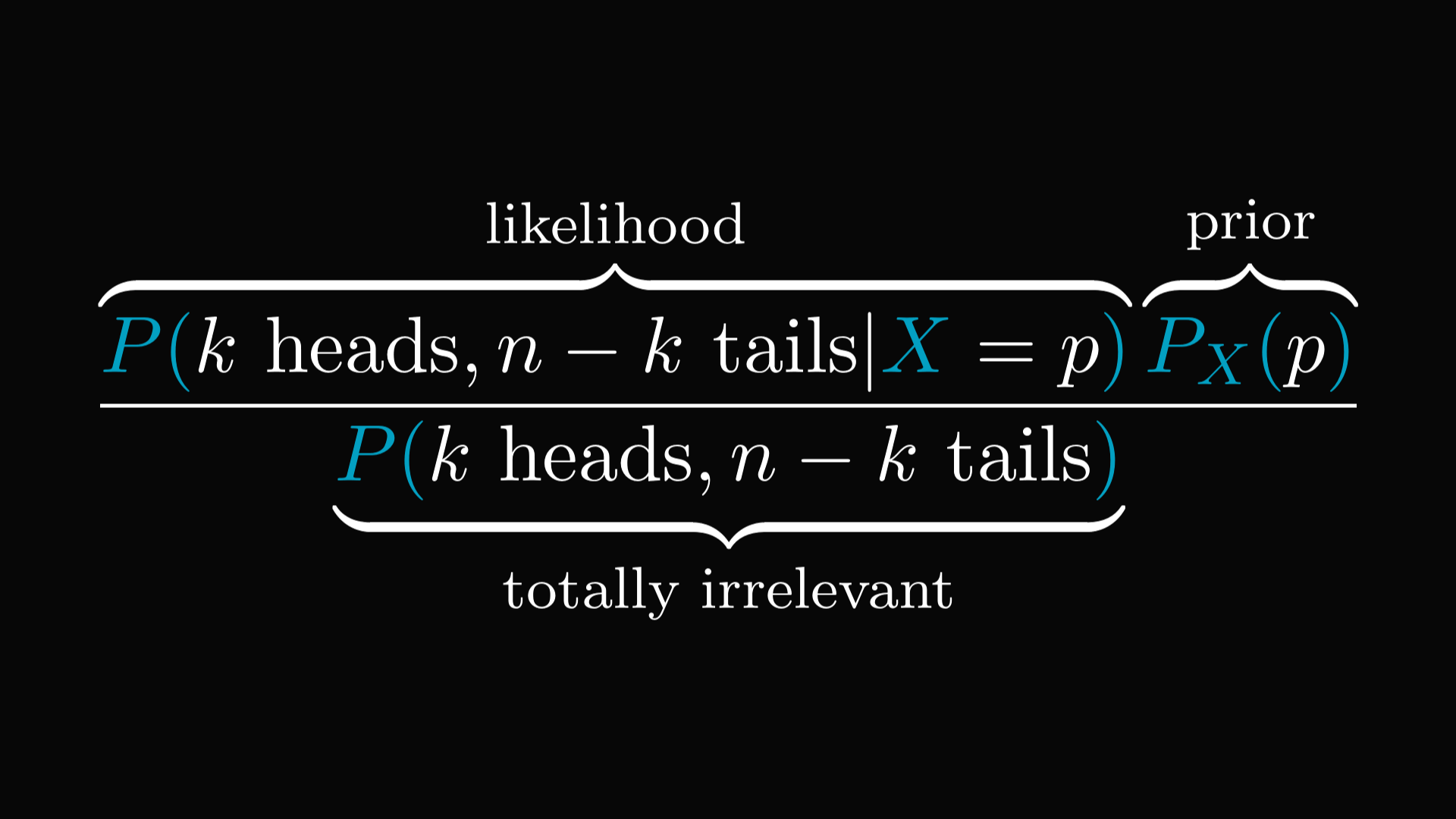Given the probability of heads, the likelihood can be computed using simple combinatorics.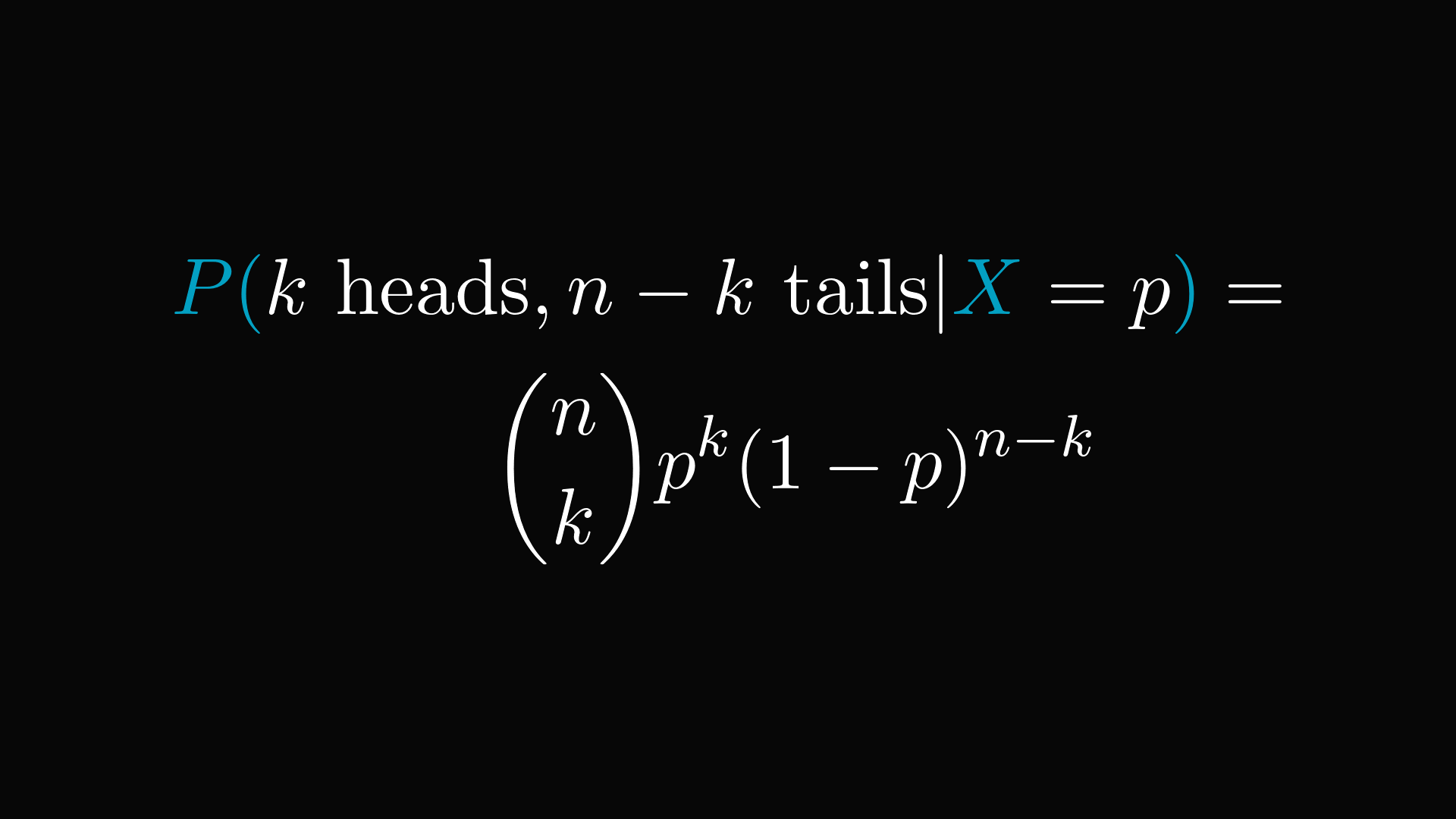After this, we get a concrete formula for the posterior density.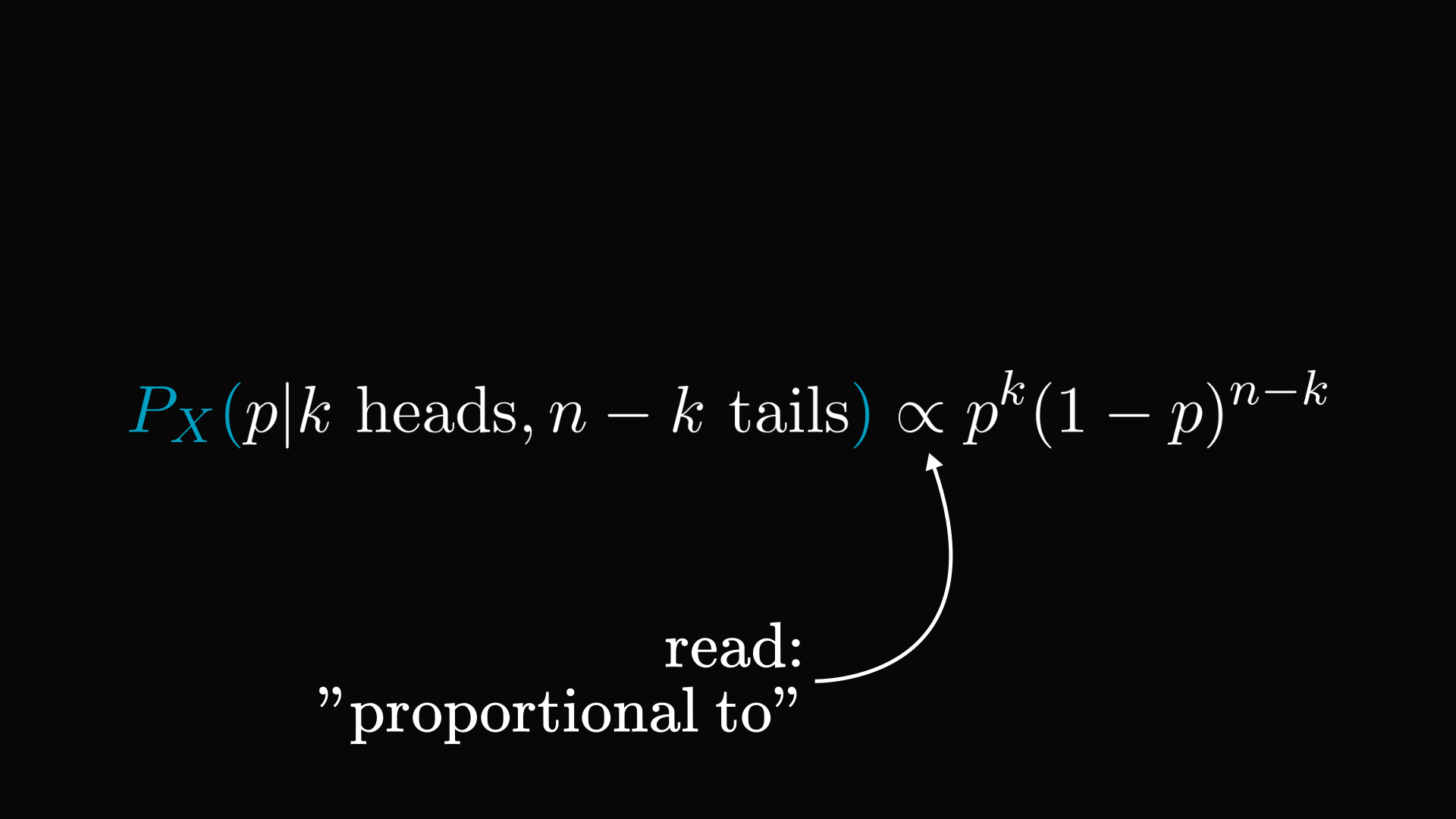To sum up: probability, as a mathematical concept, is independent of interpretation. The question of frequentist vs Bayesian comes up when we are building probabilistic models from data.

Is the Bayesian viewpoint better than the frequentist one?

No, it's just different. In certain situations, frequentist estimations are perfectly enough. In others, Bayesian methods have the advantage.

Use the right tool for the task, and don't worry about the rest.